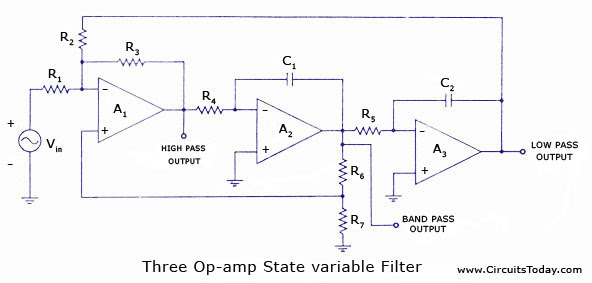## Audio

[Audio][slideshow]

With the advancement in IC technology, a number of manufacturers now offer universal filters having simultaneous low-pass, high-pass, and band-pass output responses. Notch and all-pass functions are also available by combining these output responses in the uncommitted op-amp. Because of its versatility, this filter is called the universal filter. It provides the user with easy control of the gain and Q-factor. It is also called a state-variable filter.

The filters we have discussed so far are relatively simple single op-amp circuits or several single op-amp circuits cascaded. The state-variable filter, however, makes use of three or four op-amps and two feedback paths. Though a bit more complicated, the state variable configuration offers several features not available with the other simpler filters. First, all three filter types (low-pass, band-pass, and high-pass) are available simultaneously. By properly summing these outputs some very interesting responses can be made. Bandpass filters with high Q can be built. The damping and/or critical frequency could be electroni­cally tuned.A schematic of a three op-amp, unity gain state variable filter is depicted in figure. Op-amps A2 and A3 are integrators while op-amp .A1 sums the input with the low-pass output and a portion of the bandpass output. The circuit is actually a small analog com­puter designed to solve the differential equation (transfer function) for each filter type.

For proper operation Rj = R2 = R3 = R; R4 = R5 = R,; and Cx = C2 = C.

The critical frequencies of each of the three filters are equal and is as given as

The damping is set by R6 and R7. This determines the types of low-pass and high-pass responses (Bessel, Butterworth, or Chebyshev)

α = 3 [R7 / R6+R7]

It also sets the Q and the gain of the bandpass filter

Q = 1/ α and Aband.pass = Q

The state variable filter produces the standard second-order low-pass band-pass, and high-pass responses. The critical frequencies of each are equal, and the damping is set by the feedback from the bandpass out­put. For all three outputs this damp­ing has precisely the same effect (at the same numerical values) as it did for the single op-amp filters. For low-pass and high-pass, the damp­ing coefficient of 1.414 provides a Butterworth response. Damping of 1.732 provides Bessel response, and α = 0.766 causes 3 db peaks (Chebyshev). The high-pass – 3 db frequencies are similarly shifted by the high-pass correction factor khp = 1/klp

For the band-pass section, changing the damping coefficient inversely alters the Q and gain (at critical frequency).

But the critical frequency is set by Rf and C. It is not altered by changes in the damping coefficient. This means that changes in damping only (and directly) affect the BW. So tuning of bandpass filter is very convenient. Resistor R adjusts the centre frequency only. Resistors RA and RB adjusts the BW only.

At this point, it is critical that we realize that optimum performance from all three outputs cannot be obtained simultaneously. For instance if we want maximum flatness in the passbands of low-pass and high-pass outputs, we must select a Butterworth response with α = 1.414. But a damping coefficient of 1.414 gives a Q and Af of 0.707 each. The bandpass filter will not be very selective and will attenuate even the centre frequency by 30%.

On the other hand, if Q is selected to be 20 to achieve reasonable selectivity and centre-frequency gain, the low-pass and high-pass outputs will have a damping coefficient of 0.05. This will cause a pass band peak of over 25 db. We can either optimize the bandpass output or the low-pass and high-pass outputs.

[Lights][grids]